Share
Any question? Call us at : +33 970 405 020
THE ART OF AFRICAN CRAFTS QUALITY
0
item
0.00 € VAT Incl.
Your shopping cart
Products search Advanced search

# To know the ring size ?

We have put in place our own system of international classification of sizes called ''Nanadiany size'' (N). This particular system covers all sizes of the rings around the world. Refer to the system of your country a little more bottom and take note of the equivalent of the size of your country among Nanadiany (N) to place your order
French and European size
Using the table choose the equivalent of your size and French European among Nanadiany.

Nanadiany Size = Size and French European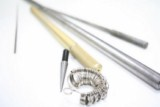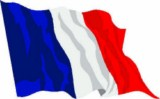Size Nanadiany N42 = Size 42 Size Nanadiany N43 = Size 43 Size Nanadiany N44 = Size 44 Size Nanadiany N45 = Size 45 Size Nanadiany N46 = Size 46 Size Nanadiany N47 = Size 47 Size Nanadiany N48 = Size 48 Size Nanadiany N49 = Size 49 Size Nanadiany N50 = Size 50 Size Nanadiany N51 = Size 51 Size Nanadiany N52 = Size 52 Size Nanadiany N53 = Size 53 Size Nanadiany N54 = Size 54 Size Nanadiany N55 = Size 55 Size Nanadiany N56 = Size 56 Size Nanadiany N57 = Size 57 Size Nanadiany N58 = Size 58 Size Nanadiany N59 = Size 59 Size Nanadiany N60 = Size 60 Size Nanadiany N61 = Size 61 Size Nanadiany N62 = Size 62 Size Nanadiany N63 = Size 63 Size Nanadiany N64 = Size 64 Size Nanadiany N65 = Size 65 Size Nanadiany N66 = Size 66 Size Nanadiany N67 = Size 67 Size Nanadiany N68 = Size 68 Size Nanadiany N69 = Size 69 Size Nanadiany N70 = Size 70 Size Nanadiany N72 = Size 72
USA and Canada size
Using the table choose the equivalent of your size from American and Canadian Nanadiany home.

Nanadiany Size = Size U.S. and Canada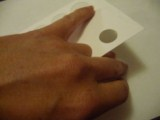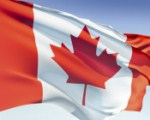English ( UK) size
Using the table choose the equivalent of your English Size (UK) among Nanadiany.

Nanadiany Size = Size English (UK)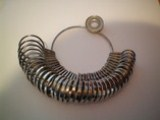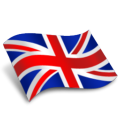German size
Using the table choose the equivalent of your German size among Nanadiany.

Nanadiany size = German size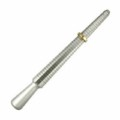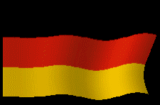Nanadiany size with the internal ring diameter in mm
To check the size: If you already have a ring that you will perfectly, measure the internal diameter and refer to the table. You will have the number that corresponds to the size of your Ring. The figure that you have chosen is the one that it must give by making a command.
Note: If however the ring received is a little too large, try putting one or several layers of nail polish (colorless or other) to the inside of the bushing in order to thicken a little. Or change of finger ... ;-)

Size Nanadiany = internal diameter of the bushing in mm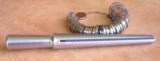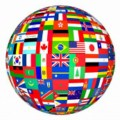N1 = 11.0 N2 = 12.1 N3 = 12.3 N4 = 12.6 N5 = 12.7 N6 = 13.0 N7 = 13.2 N8 = 13.4 N9 = 13.6 N10 = 13.8 N11 = 14.0 N12 = 14.1 N13 = 14.3 N15 = 14.5 N16 = 14.6 N17 = 14.8 N18 = 15.0 N19 = 15.2 N20 = 15.4 N49A = 15.7 N50= 15.8 N50A = 16.0 N50B = 16.1 N51 = 16.4 N51A = 16.5 N52 = 16.6 N52B = 16.7 N53 = 16.8 N53A = 17.0 N53B = 17.1 N54 = 17.2 N54A = 17.3 N55 = 17.4 N55A = 17.6 N56 = 17.7 N56A = 18.0 N57 = 18.1 N57A = 18.3 N58 = 18.5 N58A = 18.6 N59 = 18.8 N59A = 19.0 N60 = 19.1 N60A = 19.3 N61 = 19.5 N61A = 19.6 N62 = 19.8 N62A = 20.0 N63 = 20.0 N63A = 20.2 N64 = 20.3 N64A= 20.7 N65 = 20.8 N65A = 20.9 N65B = 21.1 N66 = 21.2  N67 =21.3 N68 = 21.6 N69 = 22 N70 = 22.3
Your shopping cart